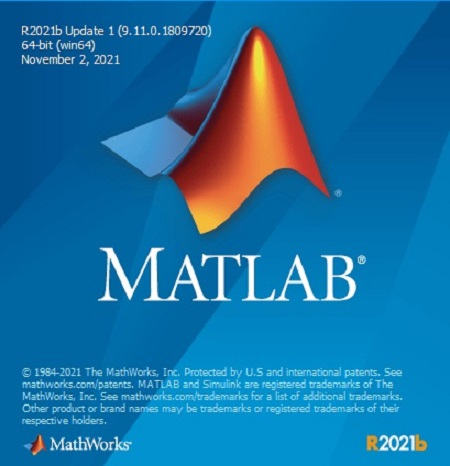﻿ MathWorks MATLAB R2021b v9.11.0.1809720 Update 1 x64 (Mac OS X) » GFXhome WS

Information of news
• Author: Mirana
• Date: 13-11-2021, 12:04
13-11-2021, 12:04

### MathWorks MATLAB R2021b v9.11.0.1809720 Update 1 x64 (Mac OS X)

Category: Software » Software MAC

MathWorks MATLAB R2021b v9.11.0.1809720 Update 1 x64 (Mac OS X)MathWorks MATLAB R2021b v9.11.0.1809720 Update 1 x64 (Mac OS X)
x64 | File Size: 16.85 GB

Description:
Company MathWorks most complete software for computational computer produces; the main program the company that actually Passport is software MATLAB (short for Mat rix Lab Oratory and means lab Matrix) is one of the most advanced software, algorithms and math and a programming language developed generation fourth is possible to visualize and plot functions and data is provided. Icon and bookmark MATLAB with the manufacturer's logo is the same as the wave equation, L-shaped membrane and special functions have been extracted. MATLAB competitors such as Mathematica, Maple and Mathcad noted.Facilities and software features MathWorks MATLAB:

-perform a variety of complex mathematical calculations and heavy
-Development environment for managing code, files, and data
-explore ways to achieve this solution
-A variety of mathematical functions for linear algebra, statistics, Fourier analysis, optimization, filtering, numerical integration and ...
-drawing two-dimensional and three-dimensional graphics functions for visualizing data as
-Design and construction of user interfaces under the programming languages ​​C ++, C or Java
-Ability to test and measure the exact functions and graphs
-the possibility of communication signal processing, image and video
-There are various Jbhabzarhay engineering companies for specific applications such as
telecommunications, control, fuzzy, estimates, statistics, data collection, simulation systems,
neural networks, probability and ...

Extract files with WinRar 5 or Latest !Site BBcode/HTML Code:
Dear visitor, you went to the site as unregistered user.